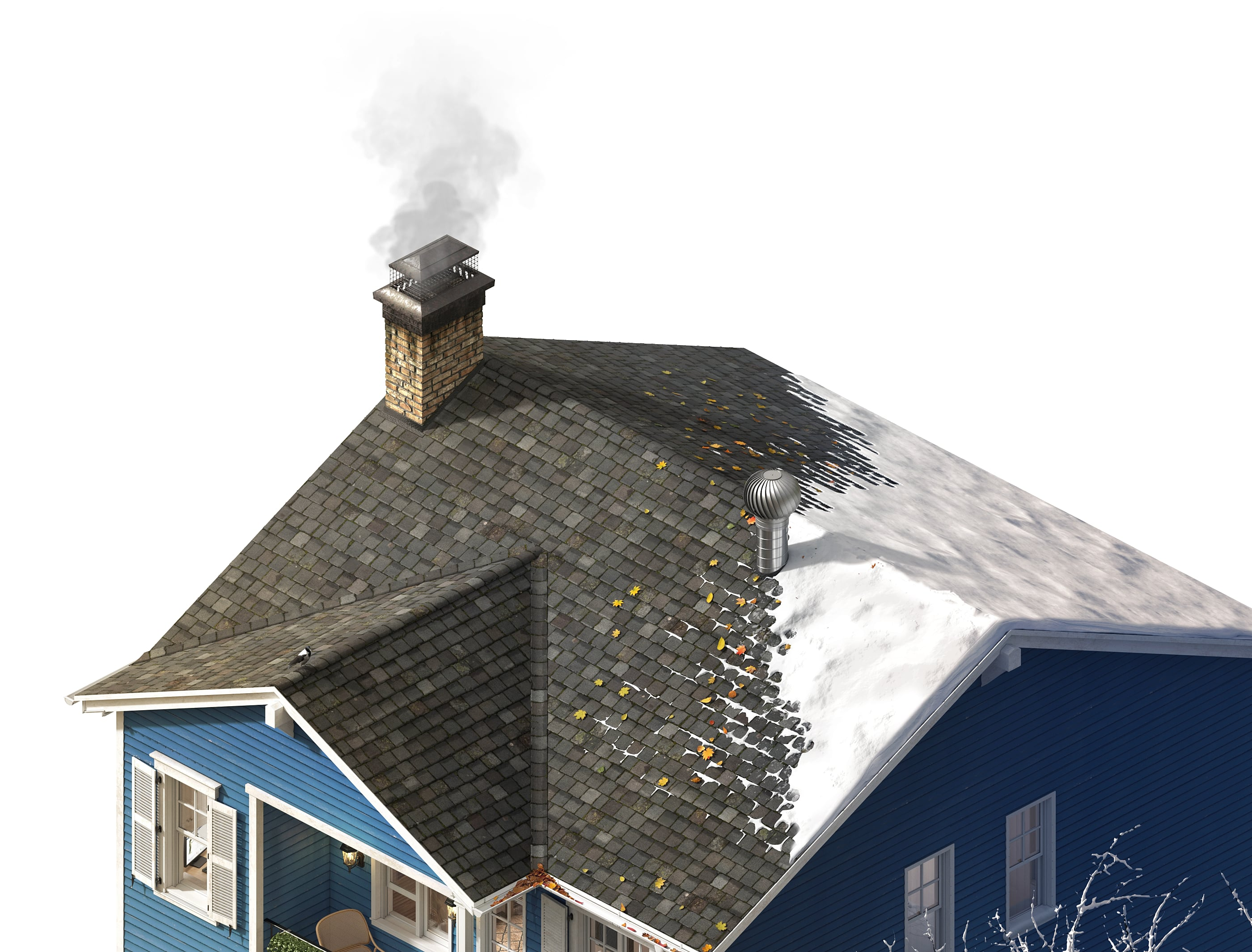Qubit House Qubit House Qubit House Qubit House Qubit House Qubit House Qubit House Qubit House Qubit House Qubit House Qubit House Qubit House Qubit House Qubit House Qubit House Qubit House Qubit House Qubit House Qubit House Qubit House Qubit House Qubit House Qubit House Qubit House Qubit House Qubit House Qubit House Qubit House Qubit House Qubit House Qubit House Qubit House Qubit House Qubit House Qubit House Qubit House Qubit House Qubit House Qubit House Qubit House Qubit House Qubit House Qubit House Qubit House Qubit House Qubit House Qubit House Qubit House Qubit House Qubit House Qubit House Qubit House Qubit House Qubit House Qubit House Qubit House Qubit House Qubit House Qubit House Qubit House Qubit House Qubit House Qubit House Qubit House Qubit House Qubit House Qubit House Qubit House Qubit House Qubit House Qubit House Qubit House Qubit House Qubit House Qubit House Qubit House Qubit House Qubit House Qubit House Qubit House Qubit House Qubit House Qubit House Qubit House Qubit House Qubit House Qubit House Qubit House Qubit House Qubit House Qubit House Qubit House Qubit House Qubit House Qubit House Qubit House Qubit House Qubit House Qubit House Qubit House Qubit House Qubit House Qubit House Qubit House Qubit House Qubit House Qubit House Qubit House Qubit House Qubit House Qubit House Qubit House Qubit House Qubit House Qubit House Qubit House Qubit House Qubit House Qubit House Qubit House Qubit House Qubit House Qubit House Qubit House Qubit House Qubit House Qubit House Qubit House Qubit House Qubit House Qubit House Qubit House Qubit House Qubit HouseHOMESICK?

HOMESICK?